• Chemistry Concept Questions and Answers## Balanced Chemical Equations Questions

Chemical equations are symbolic representations of chemical reactions that express the reactants and products in terms of their respective chemical formulae. They also use symbols to represent factors such as reaction direction and the physical states of the reacting entities.

## Balanced Chemical Equations Chemistry Questions with Solutions

Q1. A balanced chemical equation is in accordance with-

• Multiple proportion
• Reciprocal proportion
• Conservation of mass
• Definite proportions

Correct Answer: (c) Law of Conservation of Mass

Q2. The correct balanced equation for the reaction __C 2 H 6 O + __O 2 → __CO 2 + __H 2 O is-

• 2C 2 H 6 O + O 2 → CO 2 + H 2 O
• C 2 H 6 O + 3O 2 → 2CO 2 + 3H 2 O
• C 2 H 6 O + 2O 2 → 3CO 2 + 3H 2 O
• 2C 2 H 6 O + O 2 → 2CO 2 + H 2 O

Correct Answer: (b) C 2 H 6 O + 3O 2 → 2CO 2 + 3H 2 O

Q3. The correct balanced equation for the reaction __KNO 3 + __H 2 CO 3 → __K 2 CO 3 + __HNO 3 is-

• 2KNO 3 + H 2 CO 3 → K 2 CO 3 + 2HNO 3
• 2KNO 3 + 2H 2 CO 3 → K 2 CO 3 + 2HNO 3
• KNO 3 + H 2 CO 3 → K 2 CO 3 + 2HNO 3
• 2KNO 3 + 2H 2 CO 3 → K 2 CO 3 + 3HNO 3

Correct Answer: (a) 2KNO 3 + H 2 CO 3 → K 2 CO 3 + 2HNO 3

Q4. The correct balanced equation for the reaction __CaCl 2 + __Na 3 PO 4 → __ Ca 3 (PO 4 ) 2 + __ NaCl is-

• 2CaCl 2 + 2Na 3 PO 4 → 2Ca 3 (PO 4 ) 2 + NaCl
• CaCl 2 + Na 3 PO 4 → Ca 3 (PO 4 ) 2 + NaCl
• 3CaCl 2 + 2Na 3 PO 4 → Ca 3 (PO 4 ) 2 + 6NaCl
• 3CaCl 2 + 2Na 3 PO 4 → Ca 3 (PO 4 ) 2 + 3NaCl

Correct Answer- (c) 3CaCl 2 + 2Na 3 PO 4 → Ca3(PO 4 ) 2 + 6NaCl

Q5. The correct balanced equation for the reaction __TiCl 4 + __H 2 O → __TiO 2 + __HCl is-

• TiCl 4 + 2H 2 O → TiO 2 +2HCl
• TiCl 4 + 2H 2 O → TiO 2 + 4HCl
• 2TiCl 4 + H 2 O → 2TiO 2 + HCl
• TiCl 4 + 4H 2 O → TiO 2 + 4HCl

Correct Answer- (b) TiCl 4 + 2H 2 O → TiO 2 + 4HCl

Q6. Write a balanced equation for the reaction of molecular nitrogen (N 2 ) and oxygen (O 2 ) to form dinitrogen pentoxide.

Answer. The equation for the reaction is-

N 2 + O 2 → N 2 O 5 (unbalanced equation)

The balanced chemical equation is-

2N 2 + 5O 2 → 2N 2 O 5

Q7. On what basis is a chemical equation balanced?

Answer. A chemical equation is balanced using the law of conservation of mass, which states that “matter cannot be created nor destroyed.”

Q8. What is the balanced equation for the reaction of photosynthesis?

Answer. The balanced chemical equation for the reaction of photosynthesis is-

6CO 2 + 6H 2 O → C 6 H 12 O 6 + 6O 2 .

Q9. We must solve a skeletal chemical equation.” Give a reason to justify the statement.

Answer. Skeletal chemical equations are unbalanced. Due to the law of conservation of mass, we must balance the chemical equation. It states that ‘matter cannot be created or destroyed.’ As a result, each chemical reaction must have a balanced chemical equation.

Q10. What does it mean to say an equation is balanced? Why is it important for an equation to be balanced?

Answer. The chemical equation must be balanced in order to obey the law of conservation of mass. A chemical equation is said to be balanced when the number of different atoms of elements in the reactants side equals the number of atoms in the products side. Balancing chemical equations is a trial-and-error process.

Q11. What is meant by the skeletal type chemical equation? What does it represent? Using the equation for electrolytic decomposition of water, differentiate between a skeletal chemical equation and a balanced chemical equation.

Answer. Skeletal equations are those in which formulas are used to indicate the chemicals involved in a chemical reaction.

The law of conservation of mass does not apply to skeletal equations.

The chemical formulas are represented by balanced chemical equations, which follow the law of conservation of mass, which states that the atoms on the reactant and product sides are the same.

H 2 O → H 2 + O 2 : Skeletal equation

2H 2 O → 2H 2 + O 2 : Balanced chemical equation

Q12. Write the balanced chemical equation for the following reaction:

• Phosphorus burns in presence of chlorine to form phosphorus pentachloride.
• Burning of natural gas.
• The process of respiration.
• P 4 + 10Cl 2 → 4PCl 5
• CH 4 +2O 2 → CO 2 +2H 2 O + heat energy
• C 6 H 12 O 6 + 6O 2 + 6H 2 O → 6CO 2 + 12H 2 O + energy

Q13. What Is the Distinction Between a Balanced Equation and a Skeleton Equation?

Answer. The primary distinction between a balanced equation and a skeleton equation is that the balanced equation provides the actual number of molecules of each reactant and product involved in the chemical reaction, whereas a skeleton equation only provides the reactants. Furthermore, a balanced equation may or may not contain stoichiometric coefficients, whereas a skeleton equation does not.

Q14. Balance the equations

• HNO +Ca(OH) 2 → Ca(NO 3 ) 2 + H 2 O
• NaCl + AgNO 3 → AgCl + NaNO 3
• BaCl 2 +H 2 SO 4 → BaSO 4 +HCl

Answer. The balanced chemical equation for the reactions are as follows-

• 2HNO 3 + Ca(OH) 2 → Ca(NO 3 ) 2 + 2H 2 O
• BaCl 2 +H 2 SO 4 → BaSO 4 + 2HCl

Q15. Write a balanced molecular equation describing each of the following chemical reactions.

• Solid calcium carbonate is heated and decomposes to solid calcium oxide and carbon dioxide gas.
• Gaseous butane, C 4 H 10 , reacts with diatomic oxygen gas to yield gaseous carbon dioxide and water vapour.
• Aqueous solutions of magnesium chloride and sodium hydroxide react to produce solid magnesium hydroxide and aqueous sodium chloride.
• Water vapour reacts with sodium metal to produce solid sodium hydroxide and hydrogen gas.
• CaCO 3 → CaO + CO 2

On heating, 1 mol of solid calcium carbonate yields 1 mol of calcium oxide and 1 mol of carbon dioxide gas.

• 2C 4 H 10 +13O 2 → 8CO 2 + 10H 2 O When 2 moles of butane gas react with 13 moles of diatomic oxygen gas, 8 moles of carbon dioxide gas and 10 moles of water vapours are produced.
• MgCl 2 + 2NaOH → 2NaCl + Mg(OH) 2

1 mol magnesium Cordelia reacts with two moles of sodium hydroxide to produce two moles of aqueous sodium chloride solution and one mole of solid magnesium hydroxide.

• 2H 2 O + 2Na → 2NaOH + H 2

2 moles of water vapour react with 2 moles of sodium metal, yielding 2 moles of solid sodium hydroxide and 1 mol of hydrogen gas.

## Practise Questions on Balanced Chemical Equations

Balance the following equations-

1. (NH 4 ) 2 Cr 2 O 7 (s) → Cr 2 O 3 (s) + N 2 (g) + H 2 O(g)

2. Ca(OH) 2 + H 3 PO 4 → Ca 3 (PO 4 ) 2 + H 2 O

3. FeCl 3 + NH 4 OH → Fe(OH) 3 + NH 4 Cl

4. Al 2 (CO 3 ) 3 + H 3 PO 4 → AlPO 4 + CO 2 + H 2 O

5. S 8 + F 2 → SF 6

## Recommended Videos

Balancing a chemical reaction.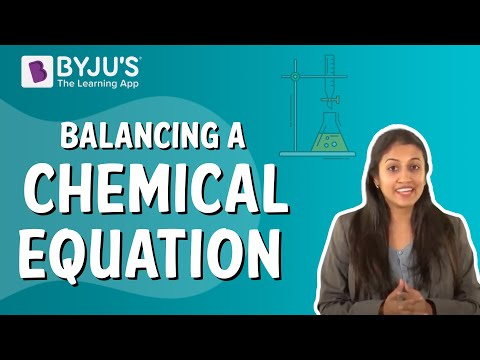## What is a Balanced Chemical Equation?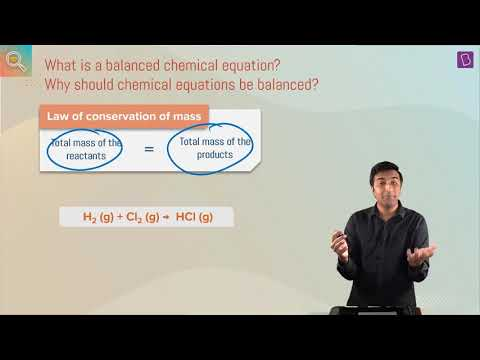Request OTP on Voice Call

Post My Comment• Share Share

Register with byju's & watch live videos.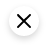## How to Balance Equations - Printable Worksheets

Balancing Equations Worksheets

• Chemical Laws
• Periodic Table
• Projects & Experiments
• Scientific Method
• Biochemistry
• Physical Chemistry
• Medical Chemistry
• Chemistry In Everyday Life
• Famous Chemists
• Activities for Kids
• Abbreviations & Acronyms
• Weather & Climate
• Ph.D., Biomedical Sciences, University of Tennessee at Knoxville
• B.A., Physics and Mathematics, Hastings College

A balanced chemical equation gives the number and type of atoms participating in a reaction, the reactants, products, and direction of the reaction. Balancing an unbalanced equation is mostly a matter of making certain mass and charge are balanced on the reactants and products side of the reaction arrow. This is a collection of printable worksheets to practice balancing equations. The printable worksheets are provided in pdf format with separate answer keys.

Balancing Chemical Equations - Worksheet #1 Balancing Chemical Equations - Answers #1 Balancing Chemical Equations - Worksheet #2 Balancing Chemical Equations - Answers #2 Balancing Chemical Equations - Worksheet #3 Balancing Chemical Equations - Answers #3 Balancing Equations - Worksheet #4 Balancing Equations - Answer Key #4

I also offer printable worksheets for balancing equations on my personal site. The printables are also available as PDF files:

Balancing Equation Practice Sheet  [ answer sheet ]​ Another Equation Worksheet [ answer sheet ] Yet Another Printable Worksheet [ answer key ]

You may also wish to review the step-by-step tutorial on how to balance a chemical equation .

## Online Practice Quizzes

Another way to practice balancing equations is by taking a quiz.

Coefficients in Balanced Equations Quiz Balance Chemical Equations Quiz

• Printable Chemistry Worksheets
• Balancing Chemical Equations
• How to Balance Chemical Equations
• Balanced Equation Definition and Examples
• Mole Relations in Balanced Equations
• Examples of 10 Balanced Chemical Equations
• 5 Steps for Balancing Chemical Equations
• What Is a Chemical Reaction?
• What Is a Chemical Equation?
• Stoichiometry Definition in Chemistry
• 20 Free Spanish Worksheets to Help Test Your Knowledge
• Introduction To Stoichiometry
• Mole Ratio: Definition and Examples
• 20 Practice Chemistry Tests
• The Balanced Chemical Equation for Photosynthesis
• Reactant Definition and Examples

By clicking “Accept All Cookies”, you agree to the storing of cookies on your device to enhance site navigation, analyze site usage, and assist in our marketing efforts.#### IMAGES

1. Balancing Chemical Equations Worksheet 2 Answer Key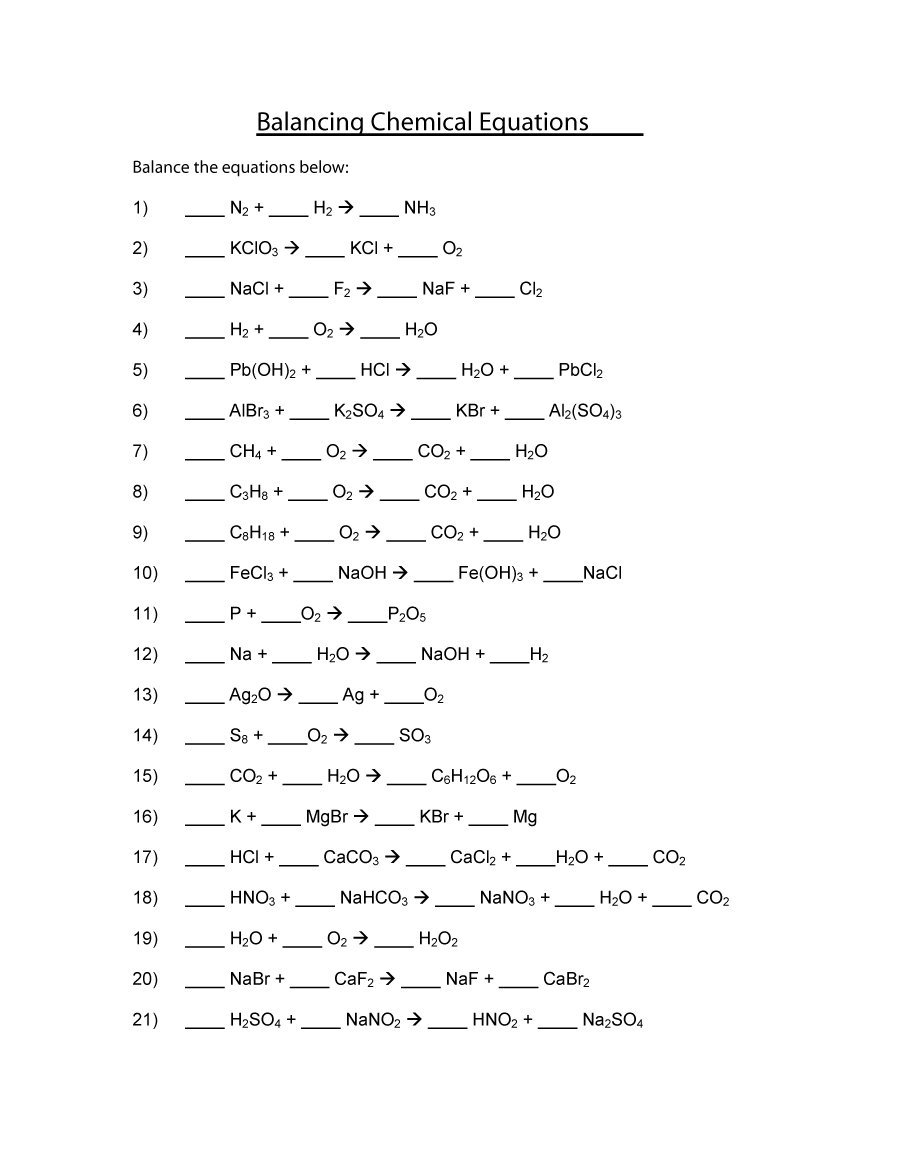2. 14 Balancing Chemical Equations Worksheet Answer Key 1-15 / worksheeto.com3. 4 Balancing Chemical Equations Worksheet Answers4. 49 Balancing Chemical Equations Worksheets [with Answers]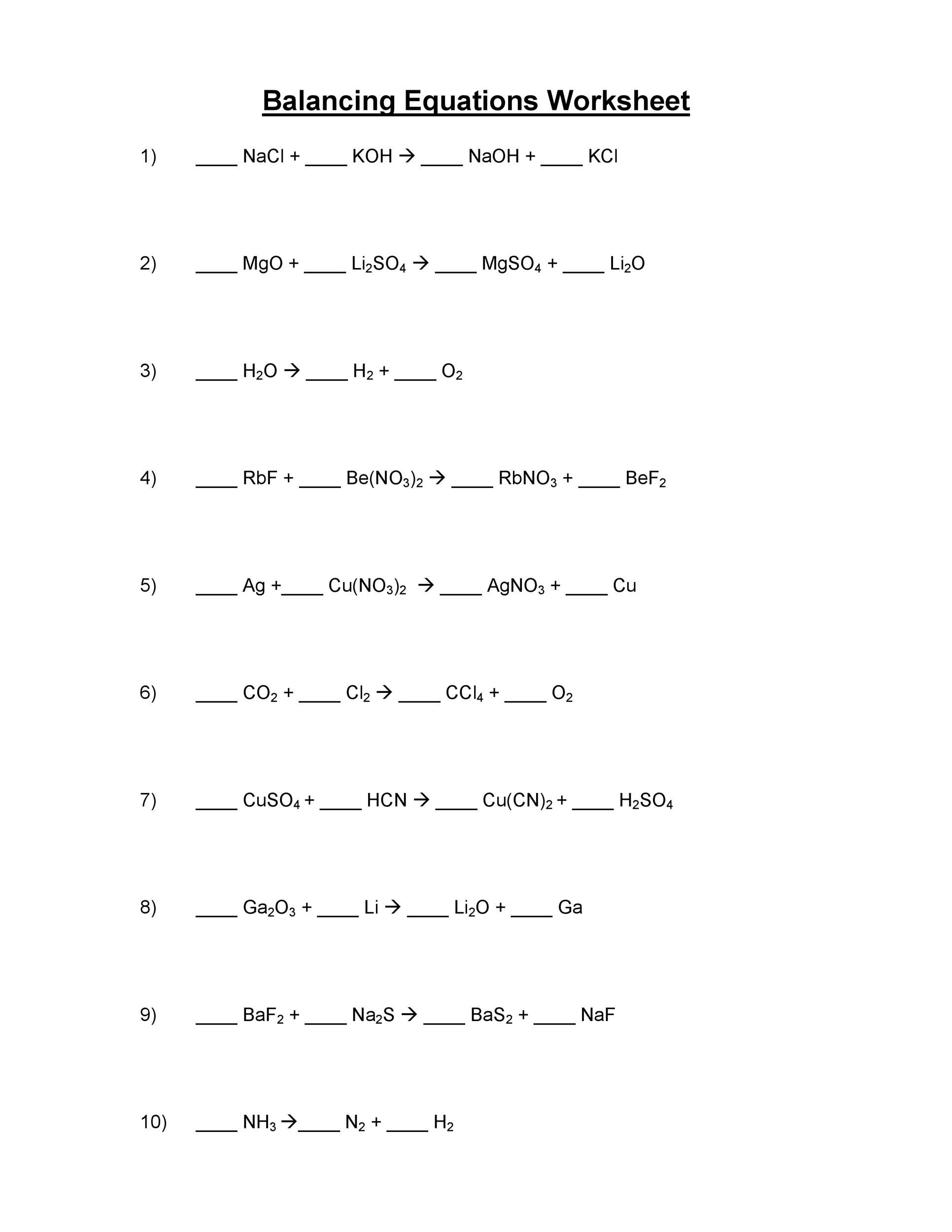5. Practice Balancing Equations #3 Answer Key6. Balancing Equation Practice Worksheet Answers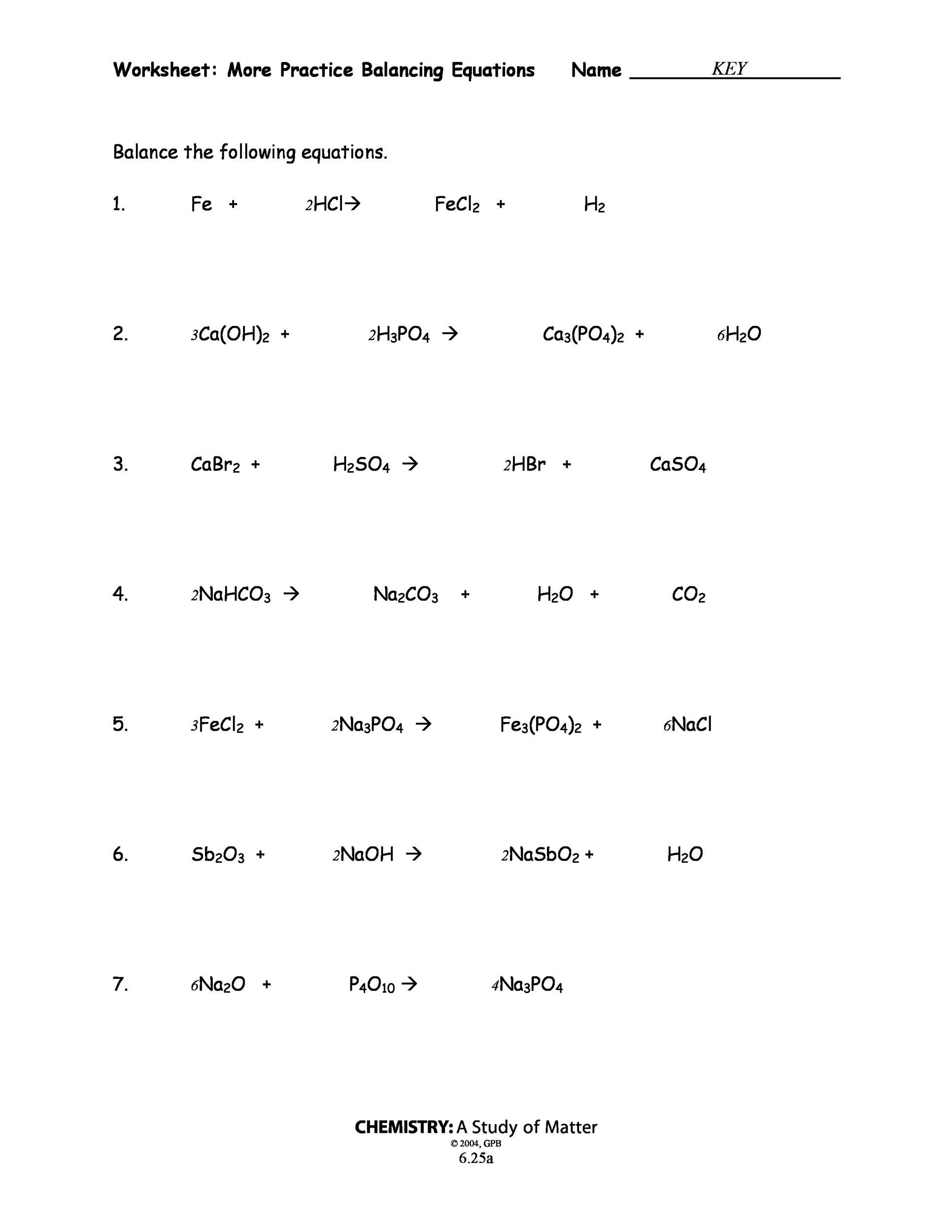#### VIDEO

1. How to balance chemical equation /Balancing chemical equation ||Class 10 Chemistry #viral #shorts

2. balancing chemical equations class10in tamil

3. Balancing of Chemical Equations

4. How to Balance Chemical Equations High School Level

5. How to balance a chemical equation ll NCERT class 10 chapter 1 chemical reactions and equations

6. Balancing of a chemical equation || Hit and trial method || Chemical equation

1. Why Is It Important to Balance Chemical Equations?

It is important to balance chemical equations because there must be an equal number of atoms on both sides of the equation to follow the Law of the Conservation of Mass. The Law of Conservation of Mass was discovered by Antoine Laurent Lavo...

2. What Is the Balanced Chemical Equation for Photosynthesis?

The balanced equation for photosynthesis is: 6CO2 + 6H2O + sunlight energy = C6H12O6 + 6O2 Photosynthesis can be represented using a chemical equation: Carbon dioxide + water + light energy gives a carbohydrate + oxygen.

3. What Is the Balanced Chemical Equation for C3H8 + O2 = CO2 + H2O?

The balanced chemical equation for C3H8 +O2 = CO2 + H2O is C3H8 +5O2 = 3CO2 +4H2O. This is the chemical reaction in which C3H8 or propane burns in air or oxygen to produce carbon dioxide and water. This chemical reaction is a complete combu...

4. Worksheet Balancing Equations

14) 1 S8 + 12 O2 → 8 SO3. 15) 6 CO2 + 6 H2O → 1 C6H12O6 + 6 O2. 16) 1 K + 1

5. 49 Balancing Chemical Equations Worksheets [with Answers]

5 What are Different Types of Chemical Equations? 6 Balancing Equations Practice Worksheet; 7 How to Balance a Chemical Equation? 8 Methods You

6. Balancing Equations Worksheet

8). ___ H3PO4 + ___ Mg(OH)2. → ___ Mg3(PO4)2 + ___ H2O. 9). ___ NaOH + ___ H2CO3 → ___ Na2CO3 + ___ H2O. 10). ___ KOH + ___ HBr → ___ KBr + ___ H2O. 11). ___

7. Balanced Chemical Equations Questions

Answer. Skeletal chemical equations are unbalanced. Due to the law of conservation of mass, we must balance the chemical equation. It states that 'matter cannot

the worksheet is shown for students to use in this class, which includes answers and.

9. Balancing Chemical Equations

8). 1 C3H8 + 5 O2 → 3 CO2 + 4 H2O. 9). 2 C8H18 + 25 O2 → 16 CO2 + 18 H2O.

10. Worksheet 6.2 Word Equations

Answers to Worksheet 6.1 Writing and Balancing Equations. 1. Write the chemical equations and balance each of the following word equations. a) Aluminum metal

11. Balancing Chemical Equations

1 S8 + 12 O2 → 8 SO3. 15). 6 CO2 + 6 H2O → 1 C6H12O6 + 6 O2. 16). 1 K + 1 MgBr

12. 100 Balanced Chemical Equations Worksheets With Answers

Oct 2, 2019 - Learn the easy way to balance any chemical equation and practice with 100 free balancing chemical equations worksheets with

13. How to Balance Equations

A balanced chemical equation gives the number and type of atoms participating in a reaction, the reactants, products, and direction of the

14. Worksheet to teach balancing equations Name

Name: ___Answer Key____. Date: 8 th. Balancing Equations Worksheet I. Balance the following chemical equations. 1. 2 K + 2 H2O → 2 KOH + H2.